# Ratios and Proportions Advanced Geometry Ratios Writing Ratios

• Slides: 20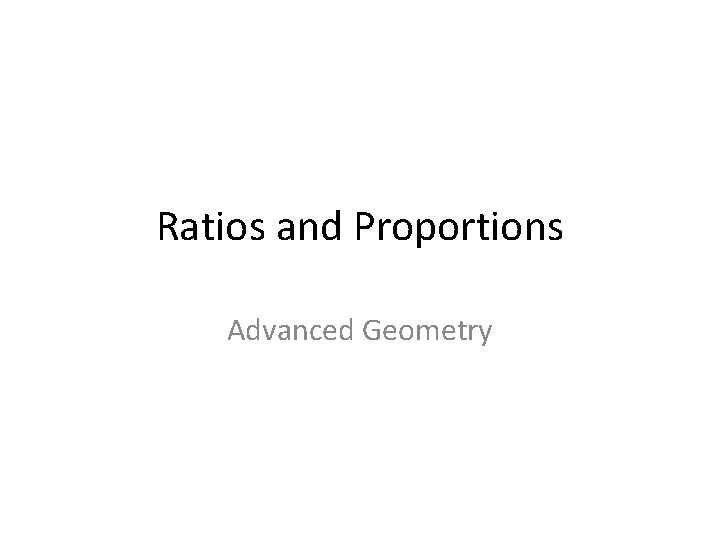Ratios and Proportions Advanced Geometry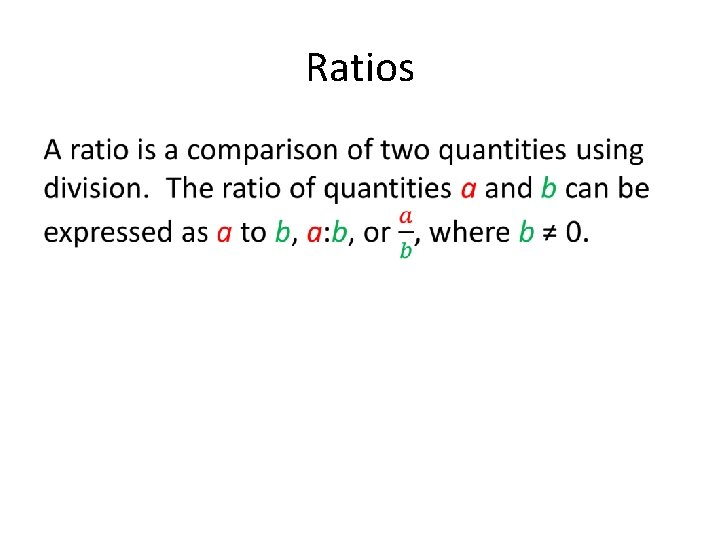Ratios •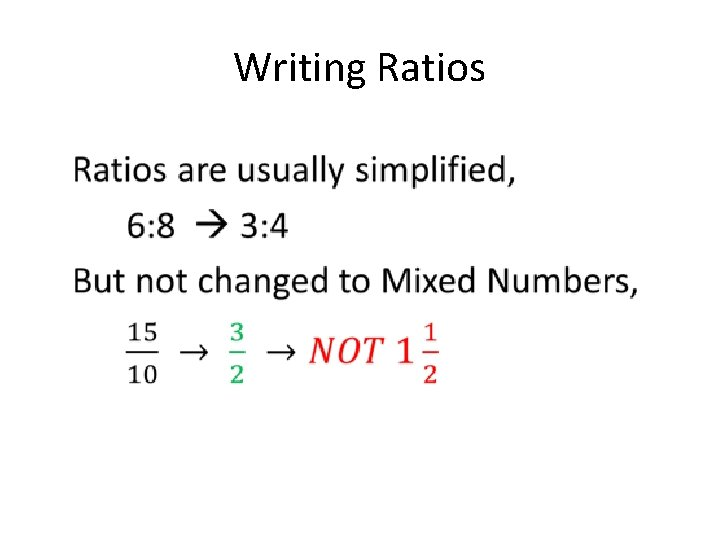Writing Ratios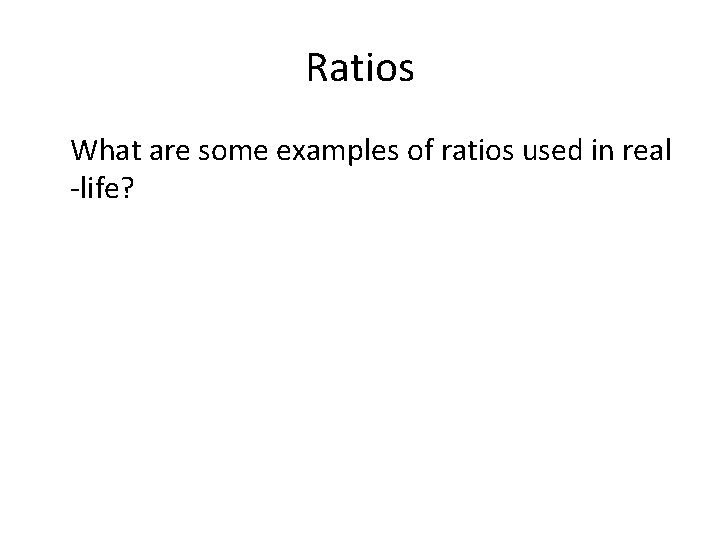Ratios What are some examples of ratios used in real -life?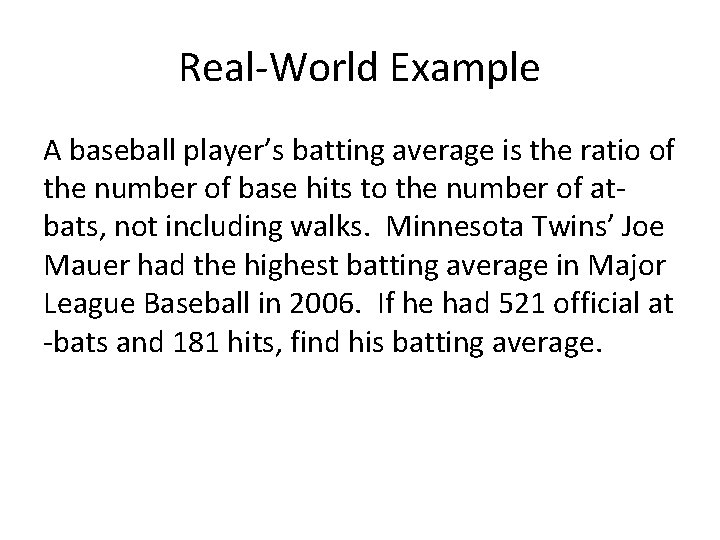Real-World Example A baseball player’s batting average is the ratio of the number of base hits to the number of atbats, not including walks. Minnesota Twins’ Joe Mauer had the highest batting average in Major League Baseball in 2006. If he had 521 official at -bats and 181 hits, find his batting average.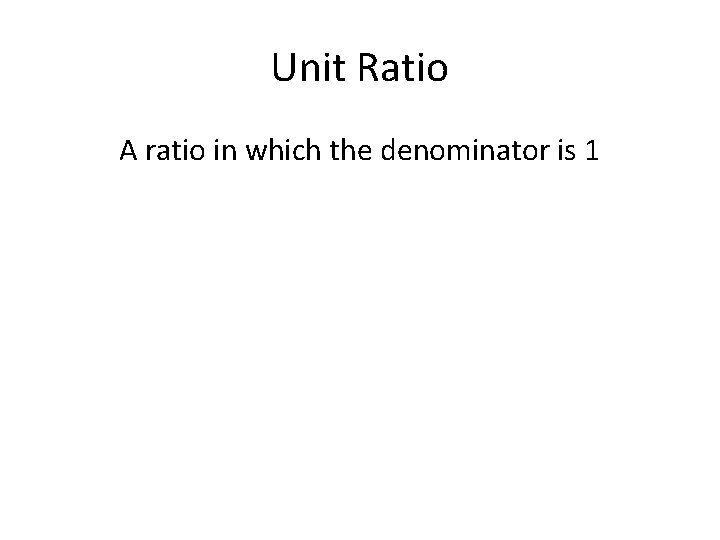Unit Ratio A ratio in which the denominator is 1Ratios about our Class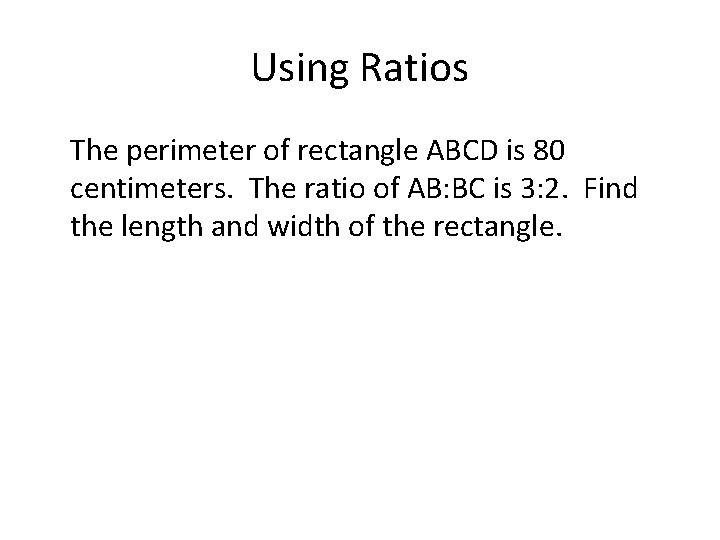Using Ratios The perimeter of rectangle ABCD is 80 centimeters. The ratio of AB: BC is 3: 2. Find the length and width of the rectangle.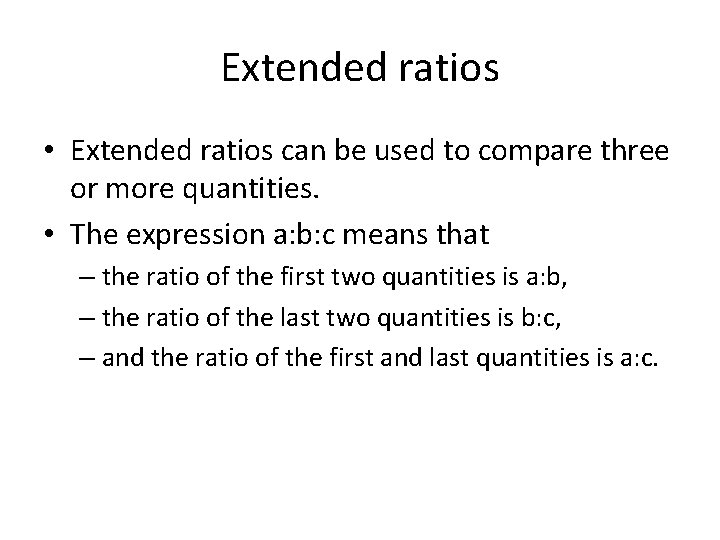Extended ratios • Extended ratios can be used to compare three or more quantities. • The expression a: b: c means that – the ratio of the first two quantities is a: b, – the ratio of the last two quantities is b: c, – and the ratio of the first and last quantities is a: c.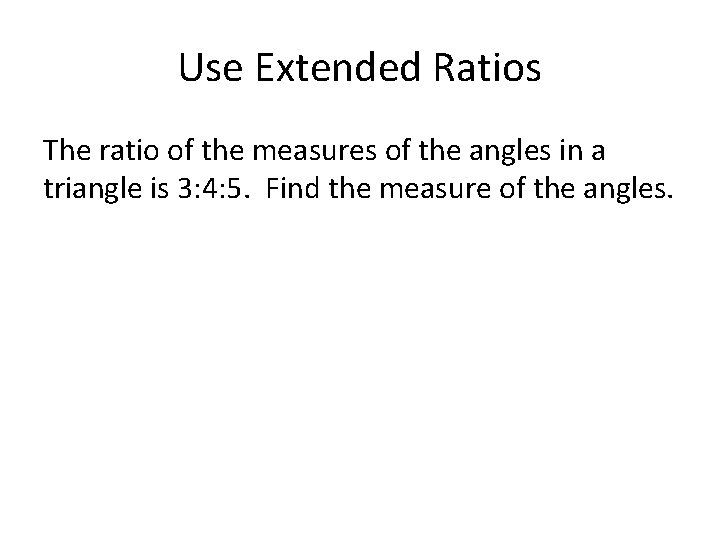Use Extended Ratios The ratio of the measures of the angles in a triangle is 3: 4: 5. Find the measure of the angles.Use Extended Ratios In a triangle, the ratio of the measures of the sides is 2: 2: 3 and the perimeter is 392 inches. Find the length of the longest side of the triangle.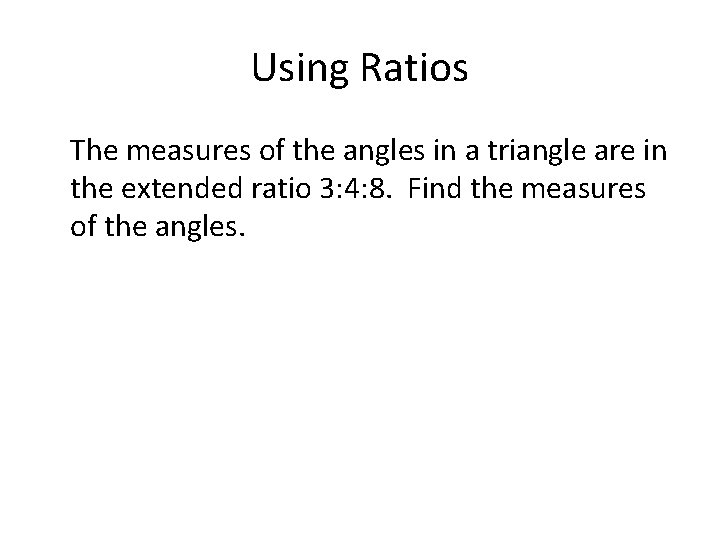Using Ratios The measures of the angles in a triangle are in the extended ratio 3: 4: 8. Find the measures of the angles.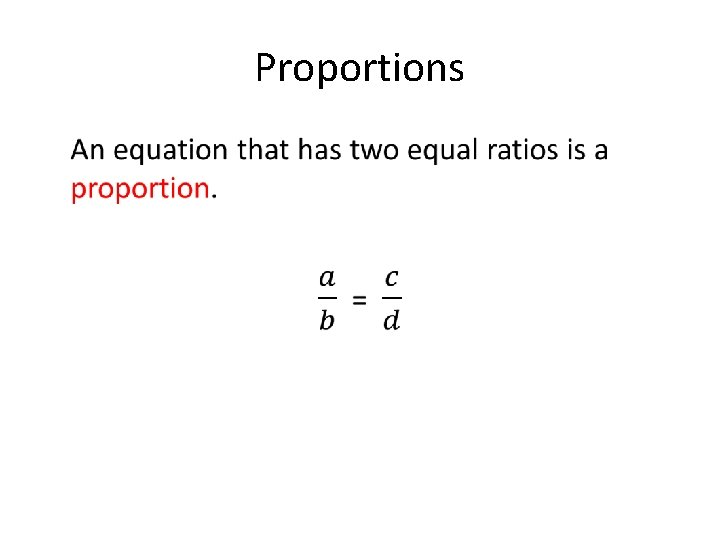Proportions •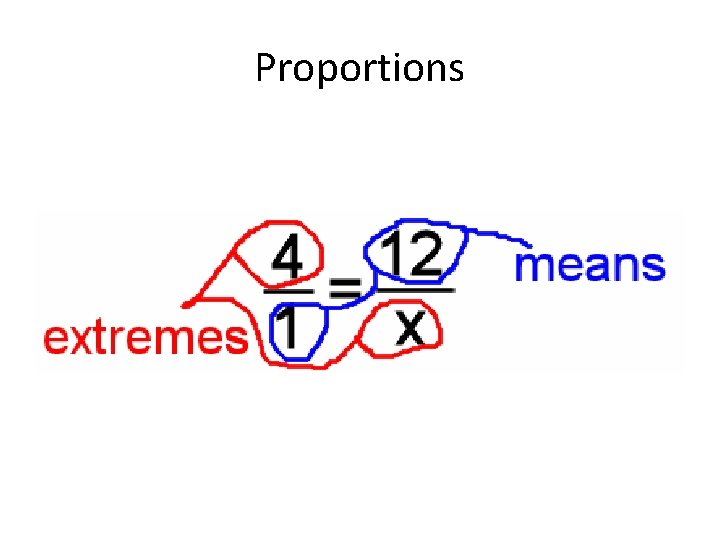Proportions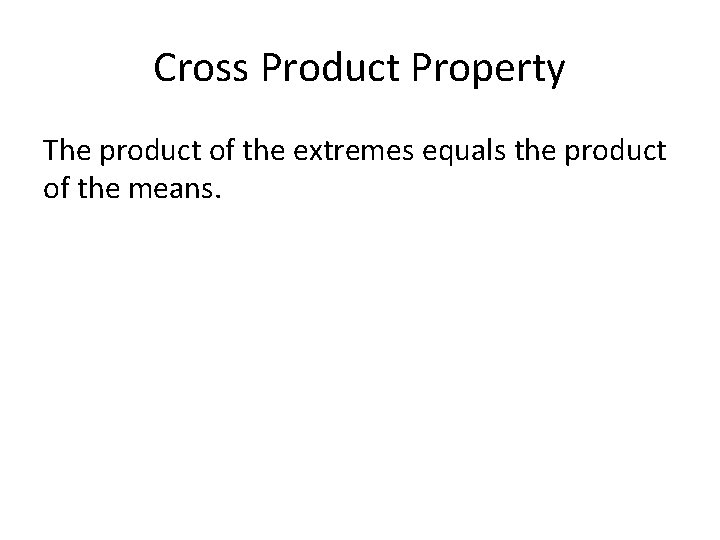Cross Product Property The product of the extremes equals the product of the means.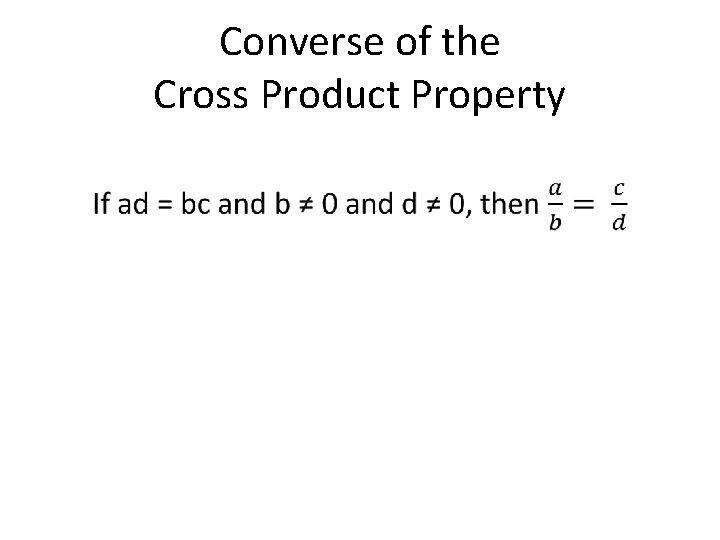Converse of the Cross Product Property •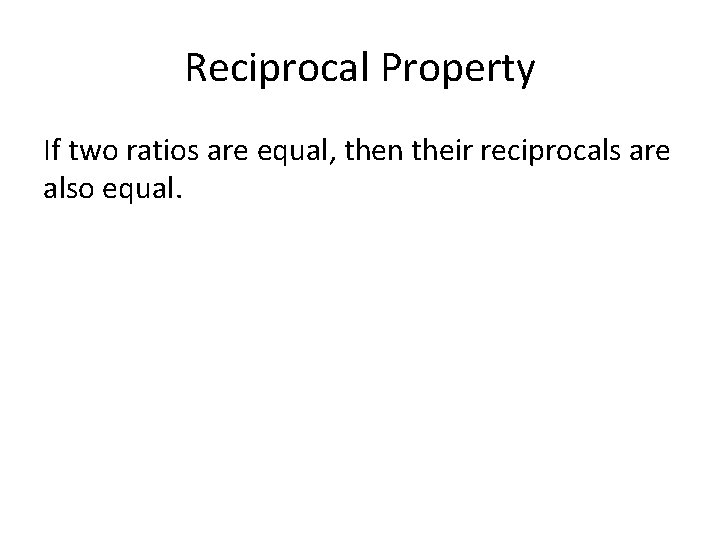Reciprocal Property If two ratios are equal, then their reciprocals are also equal.Solve Proportions •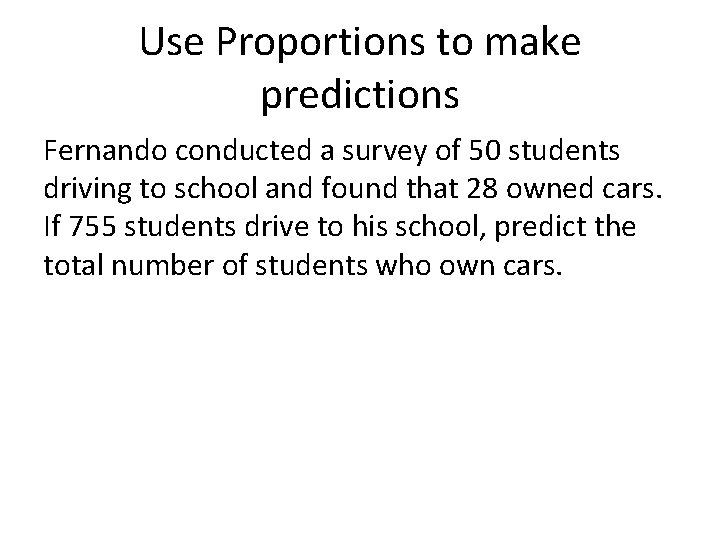Use Proportions to make predictions Fernando conducted a survey of 50 students driving to school and found that 28 owned cars. If 755 students drive to his school, predict the total number of students who own cars.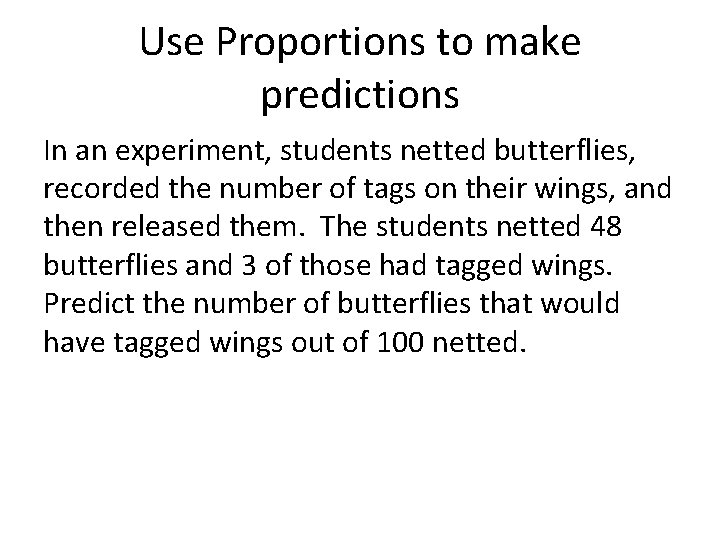Use Proportions to make predictions In an experiment, students netted butterflies, recorded the number of tags on their wings, and then released them. The students netted 48 butterflies and 3 of those had tagged wings. Predict the number of butterflies that would have tagged wings out of 100 netted.